面板数据_面板数据分析 - CSDN

• 面板数据分析方法或许我们已经了解许多了，但是到底有没有一个基本的步骤呢？那些步骤是必须的？这些都是我们在研究的过程中需要考虑的，而且又是很实在的问题。面板单位根检验如何进行？协整检验呢？什么情况下要...
转载自：http://tech.watchstor.com/storage-systems-115248.htm面板数据分析方法或许我们已经了解许多了，但是到底有没有一个基本的步骤呢？那些步骤是必须的？这些都是我们在研究的过程中需要考虑的，而且又是很实在的问题。面板单位根检验如何进行？协整检验呢？什么情况下要进行模型的修正？

面板模型回归形式的选择？如何更有效的进行回归？诸如此类的问题我们应该如何去分析并一一解决？以下是我近期对面板数据研究后做出的一个简要总结，和大家分享一下，也希望大家都进来讨论讨论。

步骤一：面板数据分析之分析数据的平稳性（单位根检验）

按照正规程序，面板数据模型在回归前需检验数据的平稳性。李子奈曾指出，一些非平稳的经济时间序列往往表现出共同的变化趋势，而这些序列间本身不一定有直接的关联，此时，对这些数据进行回归，尽管有较高的R平方，但其结果是没有任何实际意义的。这种情况称为称为虚假回归或伪回归（spurious regression）。

他认为平稳的真正含义是：一个时间序列剔除了不变的均值（可视为截距）和时间趋势以后，剩余的序列为零均值，同方差，即白噪声。因此单位根检验时有三种检验模式：既有趋势又有截距、只有截距、以上都无。

因此为了避免伪回归，确保估计结果的有效性，我们必须对各面板序列的平稳性进行检验。而检验数据平稳性最常用的办法就是单位根检验。首先，我们可以先对面板序列绘制时序图，以粗略观测时序图中由各个观测值描出代表变量的折线是否含有趋势项和（或）截距项，从而为进一步的单位根检验的检验模式做准备。

单位根检验方法的文献综述：在非平稳的面板数据渐进过程中,Levin andLin(1993) 很早就发现这些估计量的极限分布是高斯分布,这些结果也被应用在有异方差的面板数据分析中,并建立了对面板单位根进行检验的早期版本。后来经过Levin et al. (2002)的改进,提出了检验面板单位根的LLC 法。Levin et al. (2002) 指出,该方法允许不同截距和时间趋势,异方差和高阶序列相关,适合于中等维度(时间序列介于25～250 之间,截面数介于10～250 之间) 的面板单位根检验。

其中LLC-T 、BR-T、IPS-W 、ADF-FCS、PP-FCS 、H-Z 分别指Levin, Lin & Chu t* 统计量、Breitung t 统计量、lm Pesaran & Shin W 统计量、ADF- Fisher Chi-square统计量、PP-Fisher Chi-square统计量、Hadri Z统计量，并且Levin, Lin & Chu t* 统计量、Breitung t统计量的原假设为存在普通的单位根过程，lm Pesaran & Shin W 统计量、ADF- Fisher Chi-square统计量、PP-Fisher Chi-square统计量的原假设为存在有效的单位根过程， Hadri Z统计量的检验原假设为不存在普通的单位根过程。

如果我们以T（trend）代表序列含趋势项，以I（intercept）代表序列含截距项，T&I代表两项都含，N（none）代表两项都不含，那么我们可以基于前面时序图得出的结论，在单位根检验中选择相应检验模式。

并且认为，只有三个模型的检验结果都不能拒绝原假设时，我们才认为时间序列是非平稳的，而只要其中有一个模型的检验结果拒绝了零假设，就可认为时间序列是平稳的。

此外，单位根检验一般是先从水平（level）序列开始检验起，如果存在单位根，则对该序列进行一阶差分后继续检验，若仍存在单位根，则进行二阶甚至高阶差分后检验，直至序列平稳为止。我们记I(0)为零阶单整，I(1)为一阶单整，依次类推，I(N)为N阶单整。

步骤二：面板数据分析之协整检验或模型修正

情况一：如果基于单位根检验的结果发现变量之间是同阶单整的，那么我们可以进行协整检验。协整检验是考察变量间长期均衡关系的方法。所谓的协整是指若两个或多个非平稳的变量序列，其某个线性组合后的序列呈平稳性。此时我们称这些变量序列间有协整关系存在。因此协整的要求或前提是同阶单整。

但也有如下的宽限说法：如果变量个数多于两个，即解释变量个数多于一个，被解释变量的单整阶数不能高于任何一个解释变量的单整阶数。另当解释变量的单整阶数高于被解释变量的单整阶数时，则必须至少有两个解释变量的单整阶数高于被解释变量的单整阶数。如果只含有两个解释变量，则两个变量的单整阶数应该相同。

也就是说，单整阶数不同的两个或以上的非平稳序列如果一起进行协整检验，必然有某些低阶单整的，即波动相对高阶序列的波动甚微弱（有可能波动幅度也不同）的序列，对协整结果的影响不大，因此包不包含的重要性不大。

而相对处于最高阶序列，由于其波动较大，对回归残差的平稳性带来极大的影响，所以如果协整是包含有某些高阶单整序列的话（但如果所有变量都是阶数相同的高阶，此时也被称作同阶单整，这样的话另当别论），一定不能将其纳入协整检验。

协整检验方法的文献综述：

(2)Pedron(1999)在零假设是在动态多元面板回归中没有协整关系的条件下给出了七种基于残差的面板协整检验方法。和Kao的方法不同的是,Pedroni的检验方法允许异质面板的存在。

我们主要采用的是Pedroni、Kao、Johansen的方法。

通过了协整检验，说明变量之间存在着长期稳定的均衡关系，其方程回归残差是平稳的。因此可以在此基础上直接对原方程进行回归，此时的回归结果是较精确的。

这时，我们或许还想进一步对面板数据分析做格兰杰因果检验（因果检验的前提是变量协整）。但如果变量之间不是协整（即非同阶单整）的话，是不能进行格兰杰因果检验的，不过此时可以先对数据进行处理。引用张晓峒的原话，“如果y和x不同阶，不能做格兰杰因果检验，但可通过差分序列或其他处理得到同阶单整序列，并且要看它们此时有无经济意义。”

下面简要介绍一下因果检验的含义：这里的因果关系是从统计角度而言的，即是通过概率或者分布函数的角度体现出来的：在所有其它事件的发生情况固定不变的条件下，如果一个事件X的发生与不发生对于另一个事件Y的发生的概率（如果通过事件定义了随机变量那么也可以说分布函数）有影响，并且这两个事件在时间上又有先后顺序（A前B后），那么我们便可以说X是Y的原因。

考虑最简单的形式，Granger检验是运用F-统计量来检验X的滞后值是否显著影响Y（在统计的意义下，且已经综合考虑了Y的滞后值；如果影响不显著，那么称X不是Y的“Granger原因”（Granger cause）；如果影响显著，那么称X是Y的“Granger原因”。同样，这也可以用于检验Y是X的“原因”，检验Y的滞后值是否影响X（已经考虑了X的滞后对X自身的影响）。

Eviews好像没有在POOL窗口中提供Granger causality test，而只有unit root test和cointegration test。说明Eviews是无法对面板数据序列做格兰杰检验的，格兰杰检验只能针对序列组做。也就是说格兰杰因果检验在Eviews中是针对普通的序列对(pairwise)而言的。你如果想对面板数据中的某些合成序列做因果检验的话，不妨先导出相关序列到一个组中(POOL窗口中的Proc/Make Group)，再来试试。

情况二：如果如果基于单位根检验的结果发现变量之间是非同阶单整的，即面板数据中有些序列平稳而有些序列不平稳，此时不能进行协整检验与直接对原序列进行回归。但此时也不要着急，我们可以在保持变量经济意义的前提下，对我们前面提出的模型进行修正，以消除数据不平稳对回归造成的不利影响。

如差分某些序列，将基于时间频度的绝对数据变成时间频度下的变动数据或增长率数据。此时的研究转向新的模型，但要保证模型具有经济意义。因此一般不要对原序列进行二阶差分，因为对变动数据或增长率数据再进行差分，我们不好对其冠以经济解释。难道你称其为变动率的变动率？

步骤三：面板数据分析之面板模型的选择与回归

面板数据模型的选择通常有三种形式：

一种是混合估计模型（Pooled Regression Model）。如果从时间上看，不同个体之间不存在显著性差异；从截面上看，不同截面之间也不存在显著性差异，那么就可以直接把面板数据混合在一起用普通最小二乘法（OLS）估计参数。一种是固定效应模型（Fixed Effects Regression Model）。

如果对于不同的截面或不同的时间序列，模型的截距不同，则可以采用在模型中添加虚拟变量的方法估计回归参数。一种是随机效应模型（Random Effects Regression Model）。如果固定效应模型中的截距项包括了截面随机误差项和时间随机误差项的平均效应，并且这两个随机误差项都服从正态分布，则固定效应模型就变成了随机效应模型。

在面板数据分析模型形式的选择方法上，我们经常采用F检验决定选用混合模型还是固定效应模型，然后用Hausman检验确定应该建立随机效应模型还是固定效应模型。

检验完毕后，我们也就知道该选用哪种模型了，然后我们就开始回归：

在回归的时候，权数可以选择按截面加权（cross-section weights）的方式，对于横截面个数大于时序个数的情况更应如此，表示允许不同的截面存在异方差现象。估计方法采用PCSE（Panel Corrected Standard Errors，面板校正标准误）方法。Beck和Katz(1995)引入的PCSE估计方法是面板数据模型估计方法的一个创新，可以有效的处理复杂的面板误差结构，如同步相关，异方差，序列相关等，在样本量不够大时尤为有用。

以上就是对面板数据分析戏详细介绍。


展开全文• 包含的面板数据处理stata命令。包含连老师的课堂讲义适合自学。数据处理
• ## 面板数据分析步骤及流程-R语言

万次阅读 多人点赞 2016-08-25 00:03:50
面板数据模型选择及分析步骤；附R语言代码
面板数据

面板数据（Panel Data），也成平行数据，具有时间序列和截面两个维度，整个表格排列起来像是一个面板。
面板数据举例：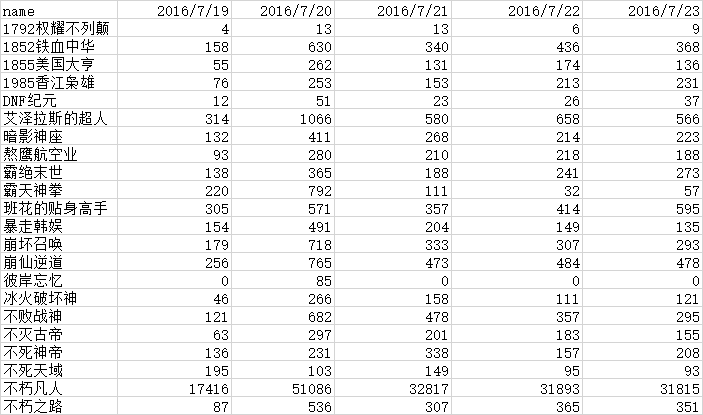模型说明及分析步骤

1、首先确定解释变量和因变量；
2、R语言操作数据格式，部分截图如下，这里以index3为因变量，index1与index2为解释变量：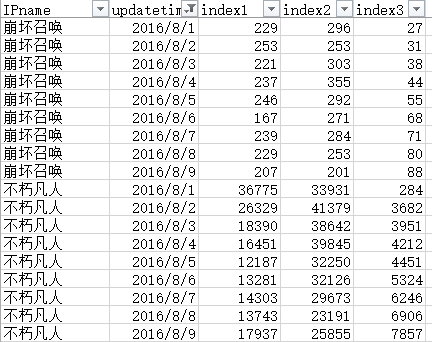##加载相关包
install.packages("mice")##缺失值处理
install.packages("plm")
install.packages("MSBVAR")
library(plm)
library(MSBVAR)
library(tseries)
library(xts)
library(mice)
data<-read.csv("F://分类别//rankdata.csv",header=T,as.is=T)##读取数据

2、单位根检验：数据平稳性

##单位根检验
tlist1<-xts(data$index1,as.Date(data$updatetime))
tlist2<-xts(data$index2,as.Date(data$updatetime))
adf.test(tlist2)

3、协整检验/模型修正
单位根检验之后，变量间是同阶单整，可进行协整检验，协整检验是用来考察变量间的长期均衡关系的方法。若通过协整检验，则说明变量间存在长期稳定的均衡关系，方程回归残差是平稳的，可进行回归。
格兰杰因果检验：前提是变量间同阶协整，通过条件概率用以判断变量间因果关系。

##格兰杰因果检验
granger.test(tsdata,p=2)

4、模型选择
面板数据模型的基本形式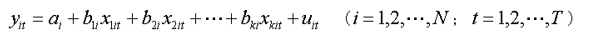也可写成：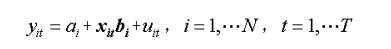其中：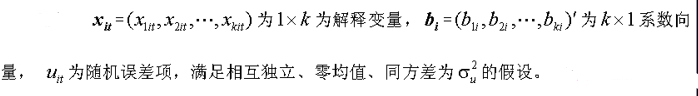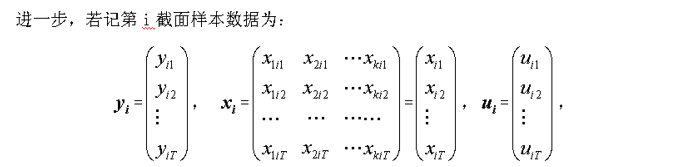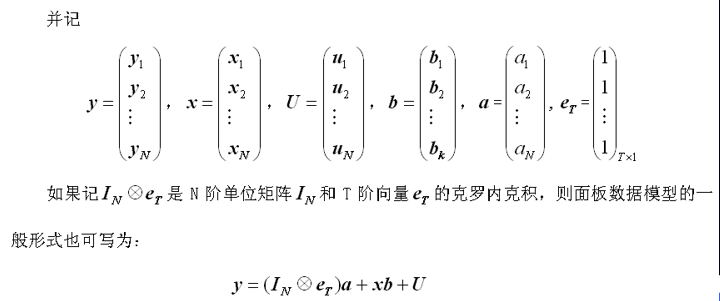对于平衡的面板数据，即在每一个截面单元上具有相同个数的观测值，模型样本观测数据的总数等于NT。
当N=1且T很大时，就是所熟悉的时间序列数据；当T=1而N很大时，就只有截面数据。

模型选择一般有三种形式
(1)无个体影响的不变系数模型（混合估计模型）：ai=aj=a，bi=bj=b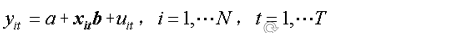即模型在横截面上无个体影响、无结构变化，可将模型简单地视为是横截面数据堆积的模型。这种模型与一般的回归模型无本质区别，只要随机扰动项服从经典基本假设条件，就可以采用OLS法进行估计（共有k+1个参数需要估计），该模型也被称为联合回归模型(pooled regression model)。
(2)变截距模型（固定效用模型）：ai≠aj，bi=bj=b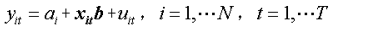即模型在横截面上存在个体影响，不存在结构性的变化，即解释变量的结构参数在不同横截面上是相同的，不同的只是截距项，个体影响可以用截距项ai (i＝1，2，…，N)的差别来说明，故通常把它称为变截距模型。
(3)变系数模型（随机效应模型）：ai≠aj，bi≠bj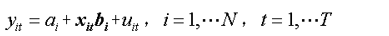即模型在横截面上存在个体影响，又存在结构变化，即在允许个体影响由变化的截距项ai (i＝1，2，…，N)来说明的同时还允许系数向量bi (i＝1，2，…，N)依个体成员的不同而变化，用以说明个体成员之间的结构变化。我们称该模型为变系数模型。
选择合适的面板模型
需要检验被解释变量yit的参数ai和bi是否对所有个体样本点和时间都是常数，即检验样本数据究竟属于上述3种情况的哪一种面板数据模型形式，从而避免模型设定的偏差，改进参数估计的有效性。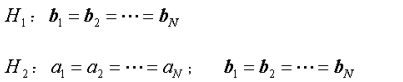如果接受假设H2，则可以认为样本数据符合不变截距、不变系数模型。如果拒绝假设H2，则需检验假设H1。如果接受H1，则认为样本数据符合变截距、不变系数模型；反之，则认为样本数据符合变系数模型。
F检验
具体计算过程略，见参考ppt。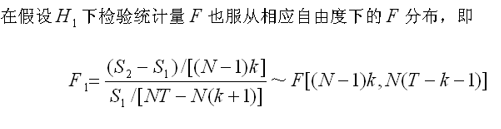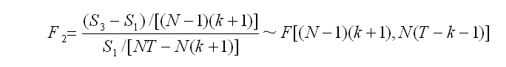其中下标1，s1指代随机效应模型的残差平方和，s2指代固定效用模型残差平方和，s3指代混合估计模型的残差平方和；
若F2统计量的值小于给定显著水平下的相应临界值，即F2小于Fa，则接受H2，认为样本数据符合混合效应模型；反之，则继续检验假设H1；
若F1统计量的值小于给定显著水平下的相应临界值，即F1小于Fa，则接受H1，认为样本数据符合固定效应模型；反之，则认为样本数据符合随机效应模型；
随机效应模型
(1)1.LM检验。Breush和Pagan于1980年提出R 检验方法。
其检验原假设和备择假设：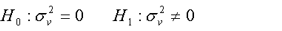如果不否定原假设，就意味着没有随机效应，应当采用固定效应模型。
(2). 豪斯曼（Hausman）检验。William H Greene于1997年提出了一种检验方法，称为豪斯曼（Hausman）检验。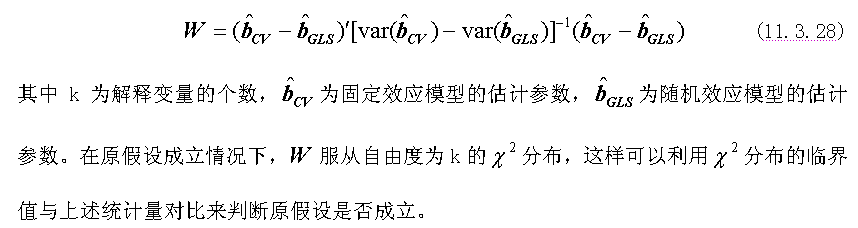若统计量大于给定显著水平下临界值，p值小于给定显著水平，则存在个体固定效应，应建立个体固定效应模型。
form<- index3~index1+ index2
rankData<-plm.data(data,index=c("IPname","updatetime"))#转化为面板数据
pool <- plm(form,data=rankData,model="pooling")#混合模型
pooltest(form,data=rankData,effect="individual",model="within")#检验个体间是否有差异
pooltest(form,data=rankData,effect="time",model="within")#检验不同时间是否有差异
wi<-plm(form,data=rankData,effect="twoways",model="within")#存在两种效应的固定效应模型
pooltest(pool,wi)#F检验判断混合模型与固定效应模型比较
phtest(form,data=rankData)##Hausman检验判断应该采用何种模型,随机效应模型检验
pbgtest(form,data=rankData,model="within")#LM检验,随机效应模型检验
#检验是否存在序列相关
pwartest(form,data=rankData)#Wooldridge检验(自相关)小于0.05存在序列相关
summary(wi)##查看拟合模型信息
fixef(wi,effect="time")#不同时间对因变量的影响程度的系数估计值
inter<-fixef(wi,effect="individual")#不同个体对因变量的影响程度的截距估计值

##根据模型参数，进行预测；

百度文库中下载的参考ppt： http://pan.baidu.com/s/1qXHVGde

注：有些地方，尤其R代码部分有些乱，需根据实际数据情况进行选择，函数的参数设置并未完全吃透，还需要继续学习，如有不对的地方，再改正，目前的理解是这样了，在本次数据场景中，实际数据应用中预测效果不是很好，误差稍大，这次未采用，以后遇到可以再尝试。
展开全文r语言 面板数据分析
• 中国335个地级市20年的面板数据，包括人口、财政等
• 面板数据(Panel Data)是将“截面数据”和“时间序列数据”综合起来的一种数据类型。具有“横截面”和“时间序列”两个维度，当这类数据按两个维度进行排列时，数据都排在一个平面上，与排在一条线上的一维数据有着...
        面板数据(Panel Data)是将“截面数据”和“时间序列数据”综合起来的一种数据类型。具有“横截面”和“时间序列”两个维度，当这类数据按两个维度进行排列时，数据都排在一个平面上，与排在一条线上的一维数据有着明显的不同，整个表格像是一个面板，所以称为面板数据(Panel Data)。

实际上如果从数据结构内在含义上，应该把Panel Data称为“时间序列-截面数据”，更能体现数据结构本质上的特点。该数据为也被称为“纵向数据(Longitudinal Data)”，“平行数据”，“TS-CS数据(Time Series-Cross Section)”。它是截面上个体在不同时间点的重复测量数据。面板数据从横截面(cross section)看，是由若干个体(entity,unit,individual)在某一时点构成的截面观测值，从纵剖面(longitudinal section)看每个个体都是一个时间序列。

从时空维度来看，可将计量经济学中应用的数据分三类：

1、横截面数据(Cross-sectional data)

横截面数据是指在某一时点收集的不同对象的数据。它对应同一时点上不同空间(对象)所组成的一维数据集合，研究的是某一时点上的某种经济现象，突出空间(对象)的差异。横截面数据的突出特点就是离散性高。横截面数据体现的是个体的个性，突出个体的差异，通常横截面数据表现的是无规律的而非真正的随机变化。即计量经济学中所谓的“无法观测的异质性”。在分析横截面数据时，应主要注意两个问题：一是异方差问题，由于数据是在某一时期对个体或地域的样本的采集，不同个体或地域本身就存在差异；二是数据的一致性，主要包括变量的样本容量是否一致、样本的取样时期是否一致、数据的统计标准是否一致。图片来源于网络

2、时间序列数据(Time-series data)

时间序列数据是指对同一对象在不同时间连续观察所取得的数据。它着眼于研究对象在时间顺序上的变化，寻找空间(对象)历时发展的规律。利用时间序列作样本时，要注意几个问题：一是所选择的样本区间内经济行为的一致性问题；二是样本数据在不同样本点之间不可比，需要对原始数据进行调整，消除其不可比因素；三是样本观测值过于集中，因而时间序列数据不适宜于对模型中反映长期变化关系的结构参数的估计；四是模型随机误差的序列相关问题。图片来源于网络

3、纵向数据(Longitudinal data)或面板数据(Panel data)

面板数据，即Panel Data，是截面数据与时间序列综合起来的一种数据资源。 在分析时,多用PanelData模型,故也被称为面板数据模型. 它可以用于分析各样本在时间序列上组成的数据的特征,它能够综合利用样本信息,通过模型中的参数,既可以分析个体之间的差异情况,又可以描述个体的动态变化特征。图片来源于网络

举栗一个，栗子来源于网络！

时间序列数据：北京一年来每天的平均温度。

截面数据：北京，上海，深圳，广州某一天的平均温度。

面板数据：北京，上海，深圳，广州这一年来每天的平均温度。

适用范围：

时间序列数据：某一个个体随时间变化产生的数据。

截面数据：许多个个体在同一个时间下由于个体不同而产生的数据。

面板数据：前两个的综合——许多个个体由于个体不同以及时间变化产生的数据。

分析方法：

时间序列数据：主要围绕时间变化，可看总体趋势，季节性，周期性，ARIMA（自回归，滑动平均，差分）等。

截面数据：主要围绕统计个体区别，可用线性回归，主元分析等。 面板数据：前两个的综合。

举栗二个，栗子来源于网络！

如：城市名：北京、上海、重庆、天津的GDP分别为10、11、9、8（单位亿元）。这就是截面数据，在一个时间点处切开，看各个城市的不同就是截面数据。

如：2000、2001、2002、2003、2004各年的北京市GDP分别为8、9、10、11、12（单位亿元）。这就是时间序列，选一个城市，看各个样本时间点的不同就是时间序列。

如：2000、2001、2002、2003、2004各年中国所有直辖市的GDP分别为：

北京市分别为8、9、10、11、12；

上海市分别为9、10、11、12、13；

天津市分别为5、6、7、8、9；

重庆市分别为7、8、9、10、11（单位亿元）。

这就是面板数据。

展开全文横截面数据 时间序列数据 面板数据
• 原始数据表，都是面板数据 目标数据表：           setwd("c:/users/11565/Desktop/合并二/可以用数据") sentiment&lt;-read.csv("001.csv") names(sentiment) dim...
原始数据表，都是面板数据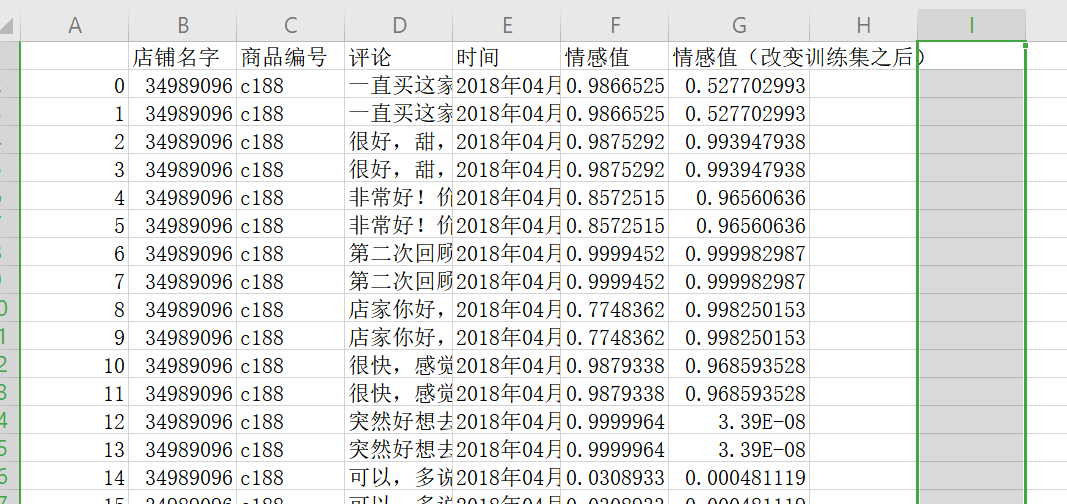目标数据表：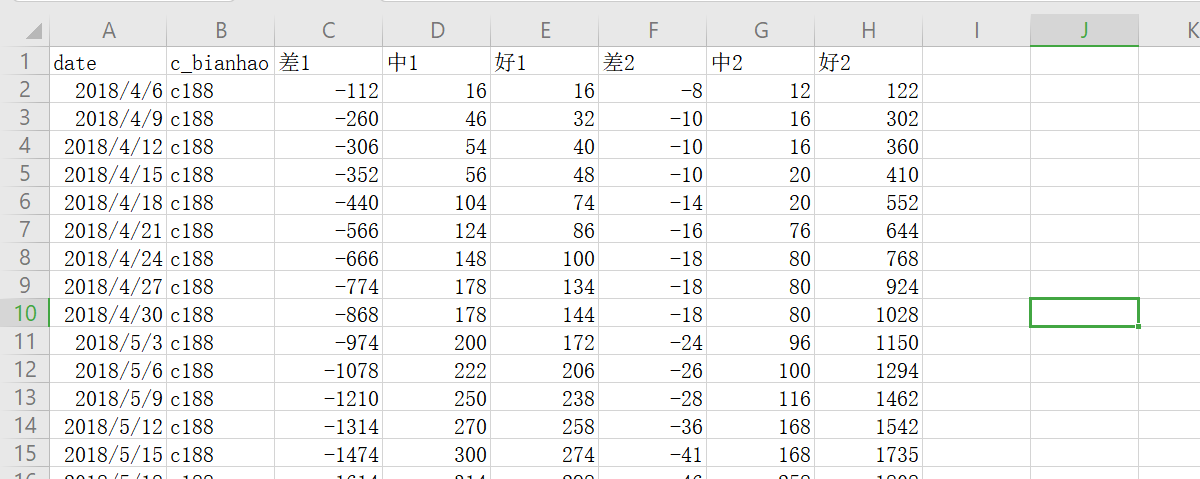setwd("c:/users/11565/Desktop/合并二/可以用数据")
names(sentiment)
dim(sentiment)
attach(sentiment)
sentiment$date<-as.character(sentiment$date)#转换成字符串
#删除含有确认的噪声数据（如确认收货之类），重复六次可以全部删除
for (j in 1:6){
for (i in (1:length(sentiment$c_bianhao))){ if ("确" %in% unlist(strsplit(sentiment$date[i], "")) == T)
sentiment=sentiment[-i,]
else{
sentiment[i,]=sentiment[i,]
}
}
}
dim(sentiment)
table(sentiment$date) sentiment<-sentiment[c(1:31600),] detach() #对sentiment_value1进行分类计算 for (i in (1:length(sentiment$c_bianhao)))
{
if (sentiment_value1[i]>=0.7)
{sentiment$critic1[i]=1 sentiment$class1[i]="好评"}
if (sentiment_value1[i]<0.7 & sentiment_value1[i]>0.3)
{sentiment$critic1[i]=2 sentiment$class1[i]="中评"}
if (sentiment_value1[i]<=0.3 && sentiment_value1[i]>0)
{sentiment$critic1[i]=-1 sentiment$class1[i]="差评"}
}
#对sentiment_value2进行分类计算
for (i in (1:length(sentiment$c_bianhao))) { if (sentiment_value2[i]>=0.7){ sentiment$critic2[i]=1
sentiment$class2[i]="好评"} if (sentiment_value2[i]<0.7 & sentiment_value2[i]>0.3) {sentiment$critic2[i]=2
sentiment$class2[i]="中评"} if (sentiment_value2[i]<=0.3 & sentiment_value2[i]>=0) {sentiment$critic2[i]=-1
sentiment$class2[i]="差评"} } detach() # write.table(sentiment,"sentiment.csv",sep=",",col.names = T,row.names = F) sentiment<-read.csv("sentiment.csv",na.strings="",stringsAsFactors = F)#已经定义好class，和去噪声的表格 x10<-table(sentiment$c_bianhao)
write.table(x10,"x10.csv",sep=",",col.names = T,row.names = F)
c_bianhao1<-x10[,1]#主要是为了保证唯一的店铺编号索引
zonghe<-data.frame("差"=c(1),"中"=c(1),"好"=c(1),"c_bianhao"=c(1),"date"=c(1))
x15=data.frame("差"=c(1,"a"),"中"=c(1,"a"),"好"=c(1,"a"),"c_bianhao"=c(1,"a"),"date"=c(0,"a"))
for (i in (1:length(c_bianhao1))){
x11<-subset(sentiment,sentiment$c_bianhao==c_bianhao1[i]) date1<-table(x11$date)
write.table(date1,"date1.csv",sep=",",col.names = T,row.names = F)
date1<-date1[,1]
for (j in (1:length(date1))){
x12<-subset(x11,x11$date == date1[j]) x13<-aggregate(x12$critic1,by=list(class=x12$class1),sum) #转换成了数据框 x13<-t(as.data.frame(x13)) colnames(x13)<-x13[1,] x13<-as.data.frame(x13) x14<-dim(x13) #是一种类型的解法 if (x14==1) { if (x13[1,1]=="差评") { x15$差=x13[,1]
x15$中=c("中评",0) x15$好=c("好评",0)
x15$c_bianhao=c(c_bianhao1[i],c_bianhao1[i]) x15$date=c(date1[j],date1[j])
zonghe<-rbind(zonghe,x15)
}else if  (x13[1,1]=="中评") {
x15$差=c("差评",0) x15$中=x13[,1]
x15$好=c("好评",0) x15$c_bianhao=c(c_bianhao1[i],c_bianhao1[i])
x15$date=c(date1[j],date1[j]) zonghe<-rbind(zonghe,x15) }else {x15$差=c("差评",0)
x15$中=c("中评",0) x15$好=x13[,1]
x15$c_bianhao=c(c_bianhao1[i],c_bianhao1[i]) x15$date=c(date1[j],date1[j])
zonghe<-rbind(zonghe,x15)
}
}else if (x14==2) {
if ((x13[1,1]=="差评") & (x13[1,2]=="中评")) {
x15$差=x13[,1] x15$中=x13[,2]
x15$好=c("好评",0) x15$c_bianhao=c(c_bianhao1[i],c_bianhao1[i])
x15$date=c(date1[j],date1[j]) zonghe<-rbind(zonghe,x15) }else if (x13[1,1]=="差评" & x13[1,2]=="好评") { x15$差=x13[,1]
x15$中=c("中评",0) x15$好=x13[,2]
x15$c_bianhao=c(c_bianhao1[i],c_bianhao1[i]) x15$date=c(date1[j],date1[j])
zonghe<-rbind(zonghe,x15)
}else{
x15$差=c("差评",0) x15$中=x13[,2]
x15$好=x13[,1] x15$c_bianhao=c(c_bianhao1[i],c_bianhao1[i])
x15$date=c(date1[j],date1[j]) zonghe<-rbind(zonghe,x15) } }else{ x15$差=x13[,1]
x15$中=x13[,2] x15$好=x13[,3]
x15$c_bianhao=c(c_bianhao1[i],c_bianhao1[i]) x15$date=c(date1[j],date1[j])
zonghe<-rbind(zonghe,x15)
}
}
}
write.table(zonghe,"综合.csv",sep=",",col.names = T,row.names = F)

#改掉语料库的淘宝店评论情感值
zonghe<-data.frame("差"=c(1),"中"=c(1),"好"=c(1),"c_bianhao"=c(1),"date"=c(1))
x15=data.frame("差"=c(1,"a"),"中"=c(1,"a"),"好"=c(1,"a"),"c_bianhao"=c(1,"a"),"date"=c(0,"a"))
for (i in (1:length(c_bianhao1))){
x11<-subset(sentiment,sentiment$c_bianhao==c_bianhao1[i]) date1<-table(x11$date)
write.table(date1,"date1.csv",sep=",",col.names = T,row.names = F)
date1<-date1[,1]
for (j in (1:length(date1))){
x12<-subset(x11,x11$date == date1[j]) x13<-aggregate(x12$critic2,by=list(class=x12$class2),sum) #转换成了数据框 x13<-t(as.data.frame(x13)) colnames(x13)<-x13[1,] x13<-as.data.frame(x13) x14<-dim(x13) #是一种类型的解法 if (x14==1) { if (x13[1,1]=="差评") { x15$差=x13[,1]
x15$中=c("中评",0) x15$好=c("好评",0)
x15$c_bianhao=c(c_bianhao1[i],c_bianhao1[i]) x15$date=c(date1[j],date1[j])
zonghe<-rbind(zonghe,x15)
}else if  (x13[1,1]=="中评") {
x15$差=c("差评",0) x15$中=x13[,1]
x15$好=c("好评",0) x15$c_bianhao=c(c_bianhao1[i],c_bianhao1[i])
x15$date=c(date1[j],date1[j]) zonghe<-rbind(zonghe,x15) }else {x15$差=c("差评",0)
x15$中=c("中评",0) x15$好=x13[,1]
x15$c_bianhao=c(c_bianhao1[i],c_bianhao1[i]) x15$date=c(date1[j],date1[j])
zonghe<-rbind(zonghe,x15)
}
}else if (x14==2) {
if ((x13[1,1]=="差评") & (x13[1,2]=="中评")) {
x15$差=x13[,1] x15$中=x13[,2]
x15$好=c("好评",0) x15$c_bianhao=c(c_bianhao1[i],c_bianhao1[i])
x15$date=c(date1[j],date1[j]) zonghe<-rbind(zonghe,x15) }else if (x13[1,1]=="差评" & x13[1,2]=="好评") { x15$差=x13[,1]
x15$中=c("中评",0) x15$好=x13[,2]
x15$c_bianhao=c(c_bianhao1[i],c_bianhao1[i]) x15$date=c(date1[j],date1[j])
zonghe<-rbind(zonghe,x15)
}else{
x15$差=c("差评",0) x15$中=x13[,2]
x15$好=x13[,1] x15$c_bianhao=c(c_bianhao1[i],c_bianhao1[i])
x15$date=c(date1[j],date1[j]) zonghe<-rbind(zonghe,x15) } }else{ x15$差=x13[,1]
x15$中=x13[,2] x15$好=x13[,3]
x15$c_bianhao=c(c_bianhao1[i],c_bianhao1[i]) x15$date=c(date1[j],date1[j])
zonghe<-rbind(zonghe,x15)
}
}
}
write.table(zonghe,"综合1.csv",sep=",",col.names = T,row.names = F)

#删除重复列
str(sentiment_1)
sentiment_2<-sentiment_1
for (i in (1:length(sentiment_1$date))){ x11<-sentiment_1$差1
if (x11[i]=="差评"){
sentiment_1<-sentiment_1[-i,]
}else
sentiment_1[i,]<-sentiment_1[-i,]
}
write.table(sentiment_1,"sentiment_1.csv",sep=",",col.names = T,row.names = F)
dim(sentiment_1)
#接下来需要对特定区间段的日期加标签，例如4月7号，4月8号，4月9号都定义在4月9号的标签
x11<-as.data.frame(table(sentiment$date)) write.table(x11,"date.csv",col.names = T,row.names = F,sep = ",")#读出来的date并没有排序 #在读date之前需要date内部的日期从小到大排列一下 x12<-read.csv("C:/Users/11565/Desktop/合并二/可以用数据/date.csv",na.strings="",stringsAsFactors = F) i=1 while (i<(length(sentiment$date)+1)){
#for (i in (1:length(sentiment$date))) j=1 while (j<(length(x12[,1])+1)){ if (sentiment$date[i]%in%(x12[,1][j:(j+2)])){
sentiment$biaoqian[i]=x12[,1][(j+2)] break } j<-j+3 } i<-i+1 } write.table(sentiment,"sentiment_2.csv",col.names = T,row.names = F,sep = ",") #将分组的数据进行加和 sentiment<-read.csv("C:/Users/11565/Desktop/合并二/可以用数据/sentiment_2.csv",na.strings="",stringsAsFactors = F) x10<-table(sentiment$c_bianhao)
write.table(x10,"x10.csv",sep=",",col.names = T,row.names = F)
c_bianhao1<-x10[,1]#主要是为了保证唯一的店铺编号索引
zonghe1<-data.frame("差1"=c(),"中1"=c(),"好1"=c(),"中2"=c(),"好2"=c(),date=c())
for (i in (1:length(c_bianhao1))){
x11<-subset(sentiment,sentiment$c_bianhao==c_bianhao1[i]) #这条命令有待商榷,是行不通的必须是选定编号商品的数据的日期,但是需要读出来以后才能进行下一步操作 #不读出来就会报错 biaoqian<-unique(x11$biaoqian)
write.table(biaoqian,"标签1.csv",sep=",",col.names = T,row.names = F)
biaoqian_1<-biaoqian1[,1]
for (j in (1:length(biaoqian_1))){
x12<-subset(x11,x11$biaoqian == biaoqian_1[j]) x13<-aggregate(x12[3:8],by=list(date=x12$biaoqian),sum)
x13$c_bianhao<-c_bianhao1[i] zonghe1<-rbind(zonghe1,x13) } } write.table(zonghe1,"sentiment_3.csv",col.names = T,row.names = F,sep = ",") #已经实现每次搜集数据时的好评数、差评数了 #累加求和 #累加求和 sentiment<-read.csv("C:/Users/11565/Desktop/合并二/可以用数据/sentiment_3.csv",na.strings="",stringsAsFactors = F) x10<-unique(sentiment$c_bianhao)
write.table(x10,"x10.csv",sep=",",col.names = T,row.names = F)
c_bianhao1<-x10[,1]#主要是为了保证唯一的店铺编号索引
#事先定义累加的函数
fd<-function(x){
for (i in 2:length(x)){
x[i]=x[i-1]+x[i]
}
x
}
zonghe1<-data.frame("差1"=c(),"中1"=c(),"好1"=c(),"中2"=c(),"好2"=c(),date=c(),c_bianhao=c())
for (i in (1:length(c_bianhao1))){
x11<-subset(sentiment,sentiment$c_bianhao==c_bianhao1[i]) if (length(x11$差1)==1){
zonghe1<-rbind(zonghe1,x11)
}else
x111<-as.data.frame(apply(x11[,2:7],2,fd))
x111$date=x11$date
x111$c_bianhao=x11$c_bianhao
zonghe1<-rbind(zonghe1,x111)
}
write.table( zonghe1,"sentiment_4.csv",col.names = T,row.names = F,sep = ",")
#sentiment_4.csv就是最后要求的表格


展开全文• install.packages(“splm”) library(splm) library(spdep) library(sp) library(Matrix) library(sf) library(spData) install.packages(“Ecdat”) data(Produc,package = “Ecdat”) library(Ecdat) ...
• 建立面板数据模型要检验被解释变量的参数和是否对所有个体样本点和时间都是常数，即检验样本数据究竟属于哪一种面板数据模型形式，从而避免模型设定的偏差，改进参数估计的有效性。主要检验如下两个假设： 如果接受...
• 转载自： 随机效应与固定效应 方差分析主要有三种模型：即固定效应模型（fixed effects model），随机效应模型（random effects model），混合效应模型（mixed effects model）。...所谓的固定、随机、混合，...
• Eviews写入面板数据① Eviews写入面板数据① Eviews写入面板数据② Eviews写入面板数据② Eviews常用面板回归模型案例实战 Eviews常用面板回归模型案例实战
• 原始数据将上传至资源。 首先打开EViews软件，按如下图操作： 01 步 02 步 03 步 04 步（这里的1，2，3表示三个不同的公司，此处需注意“？”的使用，且两变量之间需空格隔开。） 05 步（此处之后只需要将数据...简单回归分析
• 横截面数据是在同一时间，不同统计单位相同统计指标组成的数据列。横截面数据不要求统计对象及其范围相同，但要求统计的时间相同。也就是说必须是同一时间截面上的数据。例如，为了研究某一行业各个企业的产出与投入...
• 调出Database面板 配置MySQL数据源 配置H2数据库 调出Database面板   IDEA配置Database数据源需要我们在IDEA的主界面中找到View->ToolWindows->Database，如下图所示： 配置MySQL数据源   在右侧的...IDEA MySQL
• Unity-Editor 编辑Inspector面板数据保存问题使用Editor 编辑脚本，在Inspector显示自定义UI如下 代码如下 Car.csusing UnityEngine; using System.Collections;public class Car : MonoBehaviour { public int ...数据
• 一、Data Source — 数据源Grafana支持多种不同的时序数据库数据源，Grafana对每种数据源提供不同的查询方法，而且能很好的支持每种数据源的特性。Grafana官方支持以下几种数据源：...
• ## 面板回归指南

千次阅读 2019-12-02 12:01:52
面板回归指南面板数据固定效应模型随机效应模型随机还是固定？1.固定效应模型估计：2.随机效应模型估计：3.Hausman检验附录：stata中面板回归的主要命令与参数 面板数据 面板数据有两个下脚标： 不同个体i 不同时间...
• 以下的操作都是先基于huasman检验，H检验的p值大于...1、导入数据，对面板数据进行Hausman检验 这部分内容具体可参考本人的另一篇文章，就不在多做描述https://blog.csdn.net/zzzxmfj/article/details/88976277 2、...State 面板数据 量化分析
• 检查测试中得到的响应数据是否符合预期。通过响应断言控制面板，测试人员可以添加模式字符串与响应的各个域进行比较。在断言控制面板中还可以选择字符串是否与其匹配整个响应，或者仅预期响应包含模式。可以对任何...jmeter初级
• 宝塔的liunx面板确实是我用过的至今为止最好的面板，简化很多操作，不过在使用做也会遇到一些坑，本文专门记录这些遇到的坑，顺便贴上宝塔的命令 http://www.bt.cn/btcode.html 1、重启服务器后面板打不开...liunx 宝塔
• ## LabVIEW前面板控件

千次阅读 2014-09-14 21:30:40
...